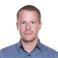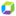cancel
Showing results for
Show  only  | Search instead for
Did you mean:

## how to find max of a series of reccordsContributor

I can use arrayMax to return a series of records each of which is the max of an array.

But now I want the max of all these records.

max(arrayMax()) and arrayMax(arrayMax())  give an error.

Here is the final part of the DQL.

| fields arrayMax(memory)

It give me these results.

"records": [
{
"arrayMax(memory)": 75.25303777058919
},
{
"arrayMax(memory)": 62.775824546813965
},
{
"arrayMax(memory)": 69.83485221862793
},
{
"arrayMax(memory)": 61.2065970102946
},
{
"arrayMax(memory)": 28.884375000000002
}
],

So how to get the max of these records?

Thank you``| summarize max(`arrayMax(memory)`)``